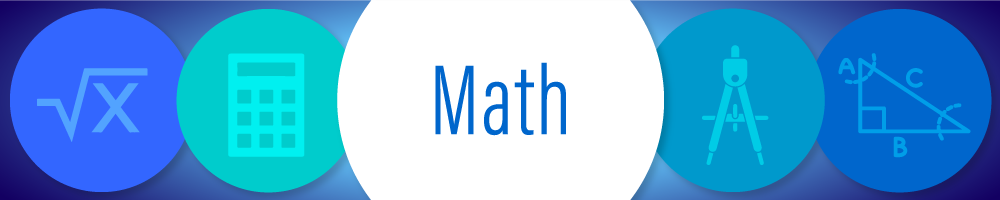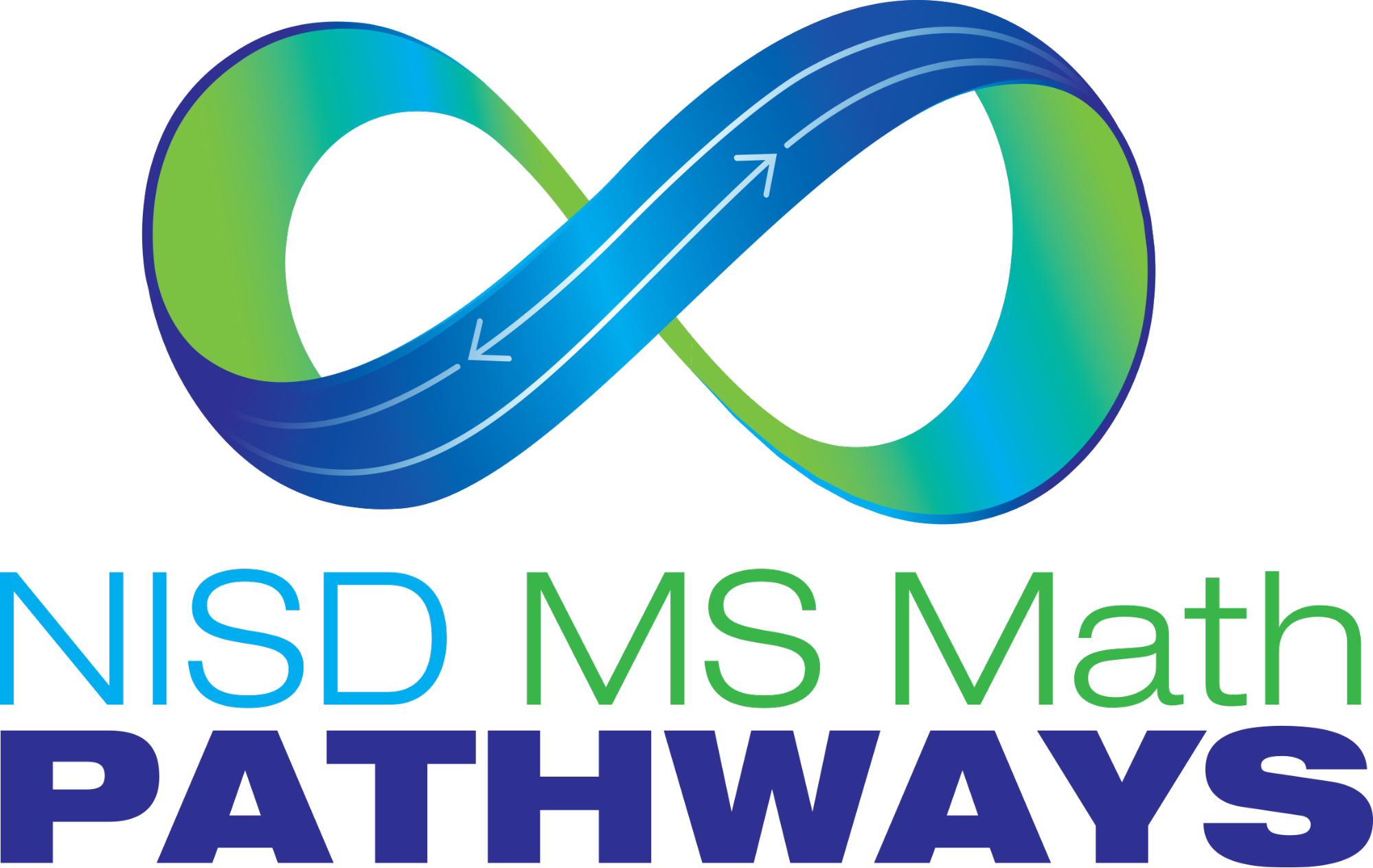# MathIn Northside ISD, we believe that all students should have access to the course pathway that will prepare them for college, career, military and beyond. To support this effort, the MS Math Program has multiple entry points from students to access Accelerated and Advanced coursework.  The courses offered in the MS Mathematics program are listed below, including a link to the Pacing Calendar for each course.### Middle School Math District Grading Policy

The district grading policy for middle school Math is as follows:

40% Formative

60% Summative

### MS Math Course Overview

 Course Name & Pacing Calendar NISD Prerequisite (or equivalent) STAAR/EOC Test Math 6 Math 5 STAAR 6 Accelerated Math 6 Math 5 STAAR 6 Math 7 Math 6 STAAR 7 MS Math Bridge Math 6 STAAR 8 Accelerated Pre-Algebra Accelerated Math 6 STAAR 8 Pre-Algebra Math 7 STAAR 8 Advanced MS Algebra Accelerated Pre-Algebra, MS Math Bridge Algebra EOC

## Math Course Descriptions

In the Math class, students will progress through the 6th Grade Math state standards (TEKS).  Topics covered include fraction and decimal operations, interpreting graphs and data, area of various polygons, proportions, percents, integers, equations, inequalities, financial literacy, and more.  Students are given support with tutoring times available. In May, students will take the 6th Grade Math STAAR test.

In the Accelerated Math class, students will progress through the curriculum at a faster pace.  By the end of the year, they will have learned all of the 6th Grade Math state standards, (TEKS), and approximately half of the 7th grade TEKS. 7th grade Accelerated Math covers the remaining 7th grade TEKS, and all of the 8th grade TEKS.  This allows students to take Advanced Algebra in 8th grade for high school credit.  Any student can register for the Accelerated 6th Grade Math class.   A teacher recommendation is not needed, just contact your child’s counselor to request it.  Students in Accelerated Math classes are given needed support and tutoring times are available.  In May, Accelerated students will take the 6th Grade Math STAAR test.

In the Math class, students will progress through the 7th Grade Math state standards (TEKS).  Topics covered include fraction and decimal operations, interpreting graphs and data, area, circumference, and volume, advanced proportions, percentages, 2-step equations, inequalities, financial literacy, and more.  Students are given support with tutoring times available. In May, students will take the 7th Grade Math STAAR test.

In the Math class, students will progress through the 8th Grade Math state standards (TEKS).  Topics covered include interpreting graphs and data, volume and surface area, financial literacy, and a heavy emphasis on Pre-Algebra topics.  Students are given support with tutoring times available. In April, students will take the 8th Grade Math STAAR test.

In Advanced Algebra, 8th grade students will progress through the state standards (TEKS) for high school Algebra I.  They will earn high school credit with successful completion of the course.  Due to the high school credit, high school requirements such as attendance (8 absences or less per semester) and semester exams will be applied.  To enroll in Advanced Algebra, students must have completed Accelerated 7th Grade Math the year before.  In May, students will take the Algebra I End-Of-Course Exam (EOC).  It is one of 5 EOC state exams students must pass as part of high school graduation requirements.

### Math SCE:

Math SCE is a support class that a student has in addition to their regular math classes.  The SCE teacher works with students to address their skill gaps from previous years and provides support to help them be successful in their current year math classes.

## Math TeachersCalendarsRegistrationHealth & Vaccination FormsBuses & BoundariesDistrict ProgramsSafeline